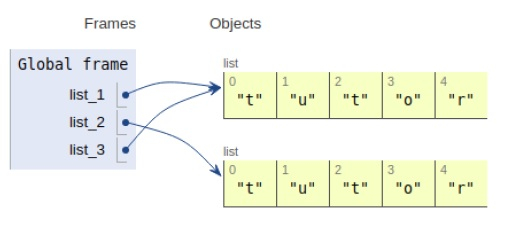# Python Object Comparison ‚Äúis‚Äù vs ‚Äú==‚Äù

In this article, we will learn about object comparision i.e. between < is > operator & equality operator < == > .

The equality operator works on the value of the passed argument whereas is operator compares the reference of the two objects passed as arguments.

In case of equality operator contents of the argument are compared ignoring their references that means the same content stored at different locations is considered identical, whereas while using is operator references are the top priority.

Now let’s observe the concept in the implementation below −

## Example

Live Demo

list_1 = ['t','u','t','o','r']
list_2 = ['t','u','t','o','r']
list_3=list_1
if (list_1 == list_2):
print("True")
else:
print("False")
if (list_1 is list_2):
print("True")
else:
print("False")
if (list_1 is list_3):
print("True")
else:
print("False")

## Output

True
False
TrueAll the variables are declared in the local scope and their references are seen in the figure above.

## Conclusion

In this article, we have learned about python object comparison by using equality & the referencing operator(is).